# Extra Hard SAT Math Question – Lost in Translation

At the end of every SAT Math section, the test makers try to come up with an extremely difficult problem that will leave even the cleverest students scratching their heads. The really evil part, though, is that even these problems can be solved in under a minute without a calculator – if you know what to do. This means that once you “figure out the trick,” these difficult problems become easy. So, while those test makers are busy cackling with sadistic glee, let’s see if we can’t beat them at their own game.

Consider the following problem:

The average of four numbers is five less than the average of the three numbers that remain after one has been eliminated. If the eliminated number is 2, what is the average of the four numbers?

A) 17

B) 22

C) 66

D) 68

E) 100

“What numbers?” you ask, “How am I supposed to find the averages of numbers that no one knows?!”

The SAT test makers love to make math questions harder by forcing you to work with variables instead of with actual numbers. Actual numbers are concrete, intuitive, and easy to understand, while variables are more abstract and difficult to grasp. At least that’s their theory. As you’ll see, variables aren’t actually harder than actual numbers. In fact, in some cases, problems that have many unknowns can actually be faster and easier to solve than problems with actual numbers, since there is less to figure out (because there’s less you can figure out). The main trick comes at the beginning, when we have to translate the words of a problem into a mathematical expression we can manipulate on our scratch paper.

First, the problem tells us that we have “the average of four numbers.” Well, how do you find the average of four numbers? You add them up and divide by 4. We can write this down if we pick variables to represent each of the four numbers. Let’s just go with a, b, c, and d.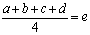Here, e represents the average of the four numbers (what we are trying to find). Next, the problem tells us that we take the average of 3 of the four numbers. This mean we have to leave out one of our variables. Since d is the last one, we’ll leave it out: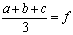The new average is represented by f. Now the problem also tells us that the variable that was left out was equal to 2, so we know that:

READ  How To Gently Kick Test Anxiety In The Face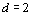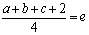The problem also tells us that the average of four numbers is five less than the average of the three numbers that remain after one has been eliminated. We have let e represent the average of the four numbers and f represent the average of the three numbers, so we could rephrase this as e is five less than f: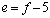So, now we still have all of these variables. We want to substitute variables for each other until we have one equation with one variable, because then we can solve for that variable and hopefully get closer to solving for e. Here’s one possible way to do that: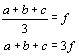We can now substitute 3f for a+b+c in the other equation: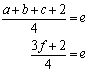We can also substitute f – 5 for e, since we know that e = f – 5: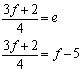Now we have one equation with only one variable, so we can solve for f: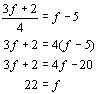Of course, we are trying to solve for e, so we should plug this into the equation e = f – 5: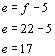Thus, the correct answer is choice A. Note, however, that if you had only solved for f, 22 was a choice as well. With this problem, it’s all about translating the words into variables and then moving the variables around until you can actually solve for one of them. This problem might be a little more time consuming than some of the others we’ve looked at in this series, but it can still be solved in under a minute without a calculator, if you know what to do. So you see, with patience and practice, even the hardest problems on the SAT become easy. As you do more practice problems you will get better and better at them – the test makers tend to use the same tricks over and over again. Check back here each week for more extra hard problems and the tricks you need to solve them! Also, remember that you can find out all the tricks from experts like me with a Test Masters course or private tutoring. Until  then, keep up the good work and happy studying!

### You Might Also Like

1.Nick says:

I’m sure you know this, but I figured out another way to solve this problem that might be easier for other people, just like it was for me. I replaced the numbers by (1x + 2x + 3x + 2 / 4) = (1x + 2x + 3x / 3) – 5, and since I had just one variable to start with, I solved for x and then replaced my x by 11 which is the answer for x, to find the average of the four numbers. So that it looked like (1(11) + 2(11) + 3(11) + 2) / 4 = (11+22+33+2) / 4 = (68)/4 = 17.

1.Bill says:

Nick,

You got the right answer and your work is mostly right, but you made an assumption that is not in the problem. There’s no way to know that the first three numbers are in the ratio of x, 2x, and 3x. You can use algebra to deduce that the sum of the first three numbers is 66, but there’s no way to know the individual values of those three numbers. In fact, it’s kind of amazing that your value of x worked out to be a whole number. However, it only worked out that way because doing it your way you get a total sum of 6x, and 66 is divisible evenly by 6.

Hope this helps!

2.Roger hitchner says:

Since the two numerators differ only by 2, it’s easier to assign a single variable, x, to the sum of the three numbers, and to solve for x:

(x+2)/4 = (x/3)-5
therefore x=66

(66+2)/4 =17

3.Chuck says:

I see a way to solve it without variables. Understanding the trick starts with knowing that the test answers provide 5 possible “averages” of the 4 numbers (17, 22, 66, 68, 100). Knowing that subtracting 2 will give you the sum of the 3 remaining numbers offers a path to reverse engineer the “average” of the 3 remaining numbers, then you can compare the averages to find a set that differ by 5.

In other words:
(1) Multiply each answer by 4 = total sum of the 4 numbers (Result: 68, 88, 264, 272, 400)
(2) Subtract 2 from the total sum = total sum of the 3 remaining numbers (Result: 66, 86, 262, 270, 398)
(3) Divide the total sum of the 3 numbers by 3 = average of the 3 numbers (only 2 of the numbers can be divided by 3 and equal an integer. Result: 22, na, na, 90, na)
(4) Compare average of 3 to average of 4: 22 is 5 more than 17; 90 is 22 more than 68. Answer is (a) 17

4.SImple says:

This is very simple actually. The method here and in comments is overcomplicated.

(x + y + t + 2)/4 = (x + y + t)/3 – 5

multiply by 12, giving:

x + y + t = 66 thus,

avg(all 4) = (66 + 2)/4 = 68/4 = 17

1.Bill says:

Simple, very good job!

5.Me Mx says:

Well i came up with really easy way .

average is total over amount .

So, Average=x/4 . I made a simple equation which was

x/4=(x-2/3)-5

Then solve X = 68 . X is sum of 4 numbers simply average is 68/4 = 17 🙂 !

Easy ?

1.Bill says:

Good job!